Next: Structure from motion Up: Computer Vision IT412 Previous: The motion field

# Optical flow

Optical flow is the apparent motion of brightness patterns in the image. Generally, optical flow corresponds to the motion field, but not always. For example, the motion field and optical flow of a rotating barber's pole are different, as illustrated in figure 3. In general, such cases are unusual, and for this lecture we will assume that optical flow corresponds to the motion field.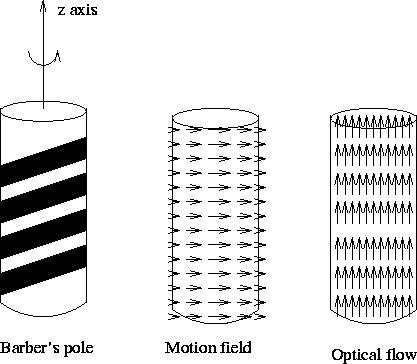One problem we do have to worry about, however, is that we are only able to measure the component of optical flow that is in the direction of the intensity gradient. We are unable to measure the component tangential to the intensity gradient. This problem is illustrated in figure 4, and further developed below.Denote the intensity by I(x,y,t). This is a function of three variables as we now have spatiotemporal variation in our signal. To see how I changes in time, we differentiate with respect to t:Now, we assume that the image intensity of each visible scene point is unchanging over time (for example, shadows and illuminations are not changing due to any motion), so we have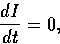which implies

Ixu + Iyv + It = 0,

where the partial derivatives of I are denoted by subscripts, and u and v are the x and y components of the optical flow vector.

This last equation is called the optical flow constraint equation since it expresses a constraint on the components u and v of the optical flow.

The optical flow constraint equation can be rewritten as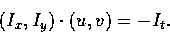Thus, the component of the image velocity in the direction of the image intensity gradient at the image of a scene point is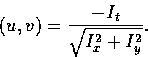We cannot, however, determine the component of the optical flow at right angles to this direction. This ambiguity is known as the aperture problem.

There are two main approaches to reconstructing three dimensional motion from motion in the image plane:

• Convert the motion problem to a stereo problem and find the correspondence between a number of points in the image at time t to the image at time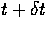.
• Computer the optical flow and use its geometrical properties to deduce three dimensional information about the scene and the motion.Next: Structure from motion Up: Computer Vision IT412 Previous: The motion field
Robyn Owens
10/29/1997